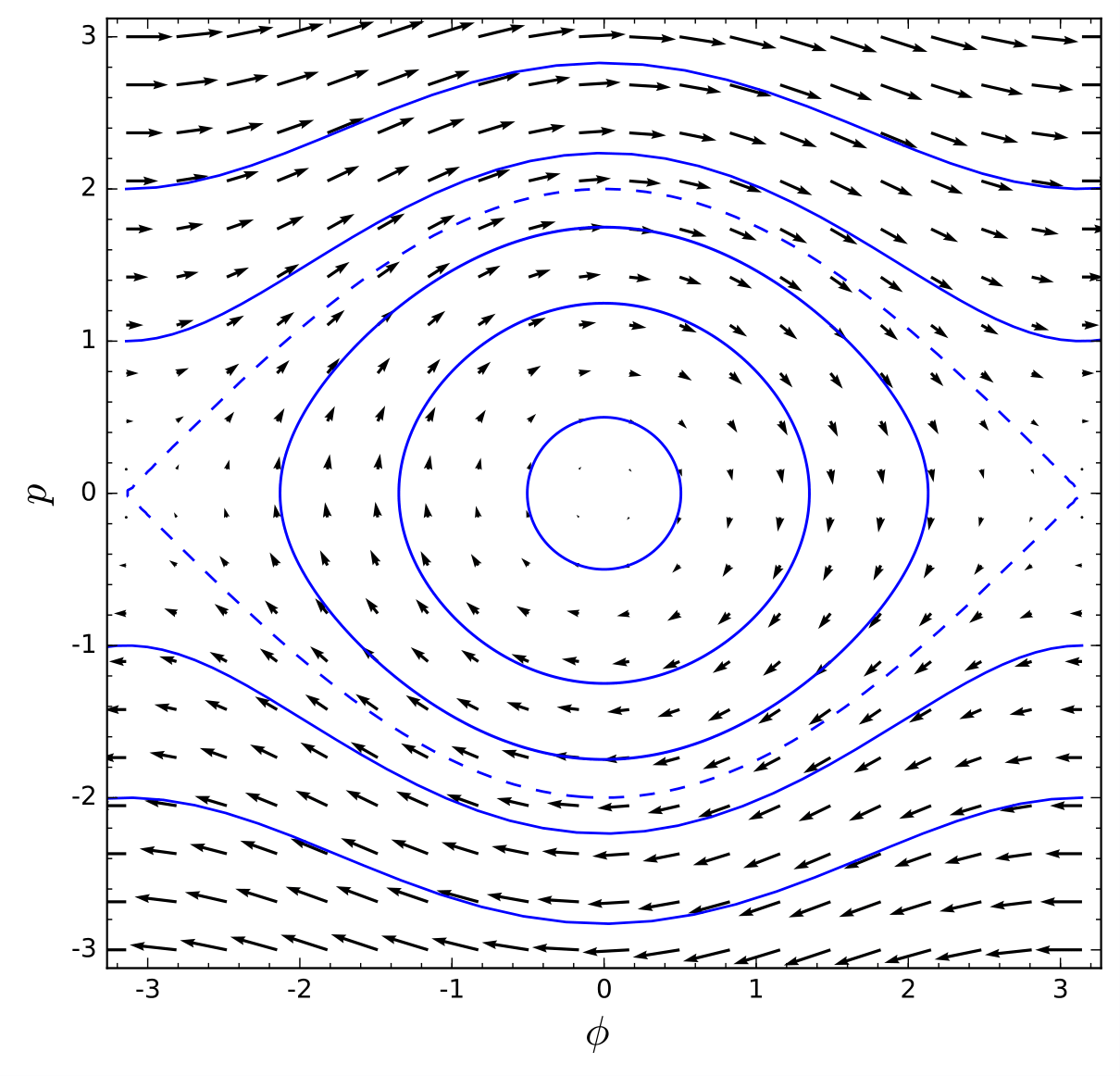# Symplectic Geometry in Classical Mechanics

During the days 9, 10 and 11 of July 2018 I taught three lessons on Symplectic Geometry in Classical Mechanics at the SEMF Summer School, organized in the Burjassot Campus at Universitat de València with the following contents:

Lesson 1: Classical Mechanics on manifolds. We start studying the Newtonian formalism and finish at the Symplectic Geometry formulation, passing through the Lagrangian Formalism. We study a practical example by constructing the configuration space on a Riemannian manifold and the phase space on its tangent bundle.

Lesson 2: Foundations of Symplectic Geometry. In this class we introduce the basic concepts of symplectic geometry: the Darboux theorem, symplectic and Hamiltonian fields and the Poisson bracket. We see how the concepts of Hamiltonian mechanics appear in a natural way in the symplectic context. Finally, we introduce the Noether theorem in its symplectic version and we study concrete examples.

Lesson 3: The theorem of Arnold-Liouville. In this class we give a proof of the theorem of Arnold-Liouville and construct the action-angle variables for general systems. After that, we study the case of harmonic oscillators and introduce conditionally periodic motion.

Here are some references:

• V.I. Arnold. Mathematical methods of Classical Mechanics. Springer-Verlag, 1989.
• H. Goldstein, C.P. Poole, and J.L. Safko. Classical Mechanichs. Pearson, 2011.
• L.D. Landau and E.M. Lifshitz. Curso de física teórica (vol. 1): Mecánica. Reverté, 1985.
• M. Spivak. Physics for Mathematicians: Mechanics I. Publish or Perish, 2010.

These are the lecture notes that I developed for the course (in Spanish):